# MINA Function

Returns the minimum value in a given list of arguments

## What is the MINA Function?

The MINA function is categorized under Excel Statistical functions. The function will return the minimum value in a given list of arguments. From a given set of numeric values, it will return the smallest value. The MINA function compares numbers, text, and logical values as TRUE or FALSE.

In financial analysis, MINA can be useful in extracting the minimum numeric value, ignoring the empty cells. For example, we can calculate the lowest test score, the slowest times in a race, the lowest temperature, or the smallest expense or sales figure.

### Formula

=MINA(value1, [value2], …)

Value1 and Value2 are the arguments used for the MINA function, where Value1 is required and the subsequent values are optional.

In MS Excel 2007 and later versions, we can provide up to 255 number arguments to the MAX function. However, in Excel 2003 and earlier versions, it can only accept up to 30 number arguments.

Arguments can be provided as constants, or as cell references or ranges. The MINA function evaluates TRUE and FALSE values as 1 and 0, respectively.

### How to use the MINA Function in Excel?

As a worksheet function, MINA can be entered as part of a formula in a cell of a worksheet. To understand the uses of the function, let us consider a few examples:

#### Example 1

Let’s calculate the lowest market expense that was expensed in the given zones.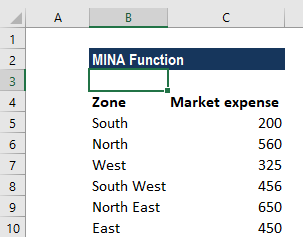The formula to use is: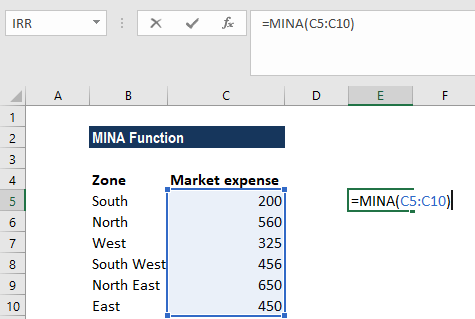We get the results below: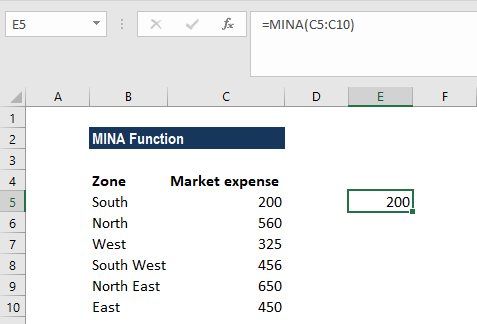#### Example 2

Suppose we are given the following data: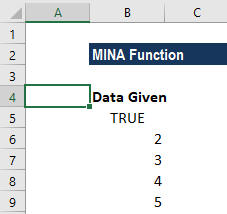Using the MINA function, we can retrieve the smallest value from the set of values in cells B5-B9.

When we give the formula: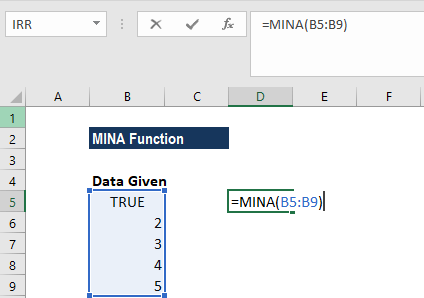We get the following result: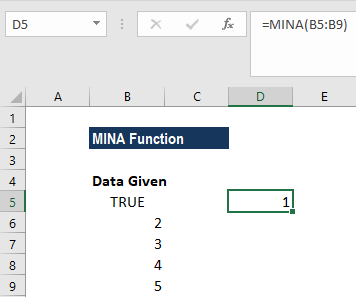We get 1 as the result. The function will treat the value TRUE in cell B5 of the spreadsheet as the numeric value 1. Therefore, it is the smallest value in the range B5-B9.

### A few things to remember about the MINA Function:

1. #VALUE! error – Occurs if any values that are supplied directly to the MINA function are non-numeric.
2. The function evaluates TRUE and FALSE values as 1 and 0, respectively.
3. The primary difference between MIN and MINA is that MINA evaluates TRUE and FALSE values as 1 and 0, respectively.

Thanks for reading CFI’s guide to important Excel functions! By taking the time to learn and master these functions, you’ll significantly speed up your financial modeling. To learn more, check out these additional CFI resources:

• Excel Functions for Finance